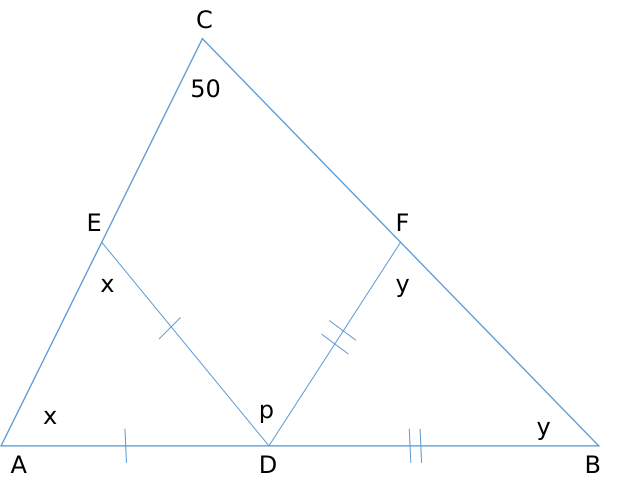Question 15

# If a triangle ABC, $$\angle BCA=50^{0}$$. D and E are points on $$AB$$ and $$AC$$, respectively, such that $$AD=DE$$. If F is a point on $$BC$$ such that $$BD=DF$$, then $$\angle FDE$$, in degrees, is equal to

SolutionWe need to find out p.

Angle ADE = 180 - 2x

Angle BDF = 180 - 2y

Now, 180 - 2y + p + 180 - 2x = 180 [Straight line = 180 deg]

p = 2x + 2y - 180

Also, x + y + 50 = 180 [Sum of the angles of triangle = 180]

x + y = 130

p = 260 - 180 = 80 degrees.

OR